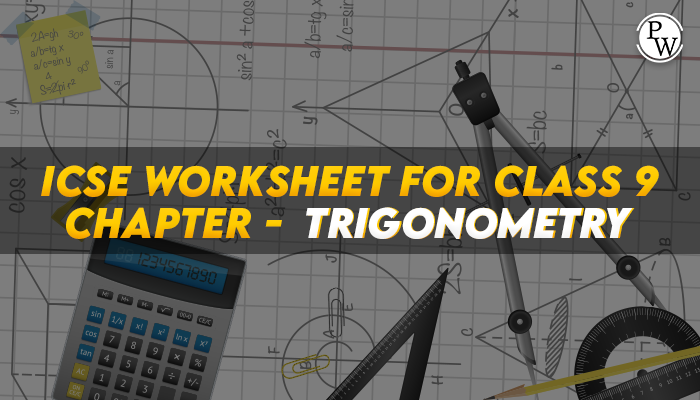# ICSE Worksheet for chapter-3 Trigonometry class 9

#### Worksheet For class 9

Physics Wallah Solutions are considered very useful when preparing for the ICSE Class 9 Maths exams. Here, we bring you the Worksheet Solutions for Class 9 Maths, providing detailed explanations of the questions in Trigonometry exercises. The subject matter experts of Physics Wallah have devised these answers as per the syllabus prescribed by the ICSE.

Physics Wallah has provided the complete solutions of Class 9 Maths Worksheet - 3 Trigonometry in PDF format. You can avail these solutions and download them for free to practice them offline. For other ICSE Worksheets for class 9 Maths, check out the main page of Physics Wallah.## Questions

Q1. If tan⁡ θ= a/b then value of is (a sinθ+b cosθ)/(a sinθ-bcosθ)=

1. (a2+b2)/(a2-b2 )
2. (a2 -b2)/(a2+b2)
3. a/√(a2+b2 )
4. 1/√(a2 +b2 )

Q2. The value of the expression 4/3 cot2 300+ 3sin2 300-2cosec2 600- 3/4 tan2 300 is

1. 1
2. (-20)/3
3. 10/3
4. 5

Q3. The value of the expression(5 sin2 300+cos2 450+4tan2 600)/(2sin300 cos600+tan450 ) is

1. 4
2. 9
3. 53/12
4. 55/6

Q4. The value of tan 50 tan 100 tan 150 tan 200……… tan 850 is

1. 1
2. 2
3. 3
4. None of these

Q5. If α+β = and sin α = then sin β is

1. √2/3
2. 2√2/3
3. 2/3
4. 3/4

Q6. If 7sinα =24 cos α; 0 < α < π/2,then value of 14 tan α -75 cos α-7sec α is equal to

1. 1
2. 2
3. 3
4. 4

Q7. If tan θ =4, then (tan⁡θ/((sin3 θ)/cosθ+sinθcosθ))is equal to

1. 0
2. 2
3. 2√2
4. 1

Q8. The area of the triangle is 12 sq.cm Two sides are 6 cm and 12cm. The included angle is:

1. cos-1 (1/3)
2. cos-1 (1/6)
3. sin-1 (1/6)
4. sin-1(1/3)

Q9. An aero plane when flying at a height 2500 m from the ground passes vertically above another aero plane. At an instant when the angles of elevation of the two aero planes from the same point on the ground are 450 ,and 300 respectively. Then the vertical distance between the two aero planes at the instant is

1. 1158 m
2. 1058 m
3. 1008 m
4. None

Q10. The shadow of a tower is 30 m when sun’s altitude is 300. When the sun’s altitude is 600, then the length of the shadow will be

1. 60 m
2. 15 m
3. 10 m
4. 5 m

Q11. The angle of elevation of a cloud from a point 200 m above a lake is 300 and the angle of depression of its reflection in the lake is 600, then the height of the cloud (in m) above the lake is

1. 200
2. 300
3. 400
4. None

Q12. The angle of elevation of the top of a vertical tower from two points 30 m apart and on the same straight line passing through the base of the tower are 300 and 600 respectively. The height of the tower is

1. 10 m
2. 15 m
3. 12m
4. 30 m

Q13. The angle of elevation of the top of tower from the top and bottom of the building h meter high are α and β then the height of the tower is

1. h sin α cos β/ sin (α+β)
2. h cos α cos β /sin (β-α)
3. h cos α sin β/sin (β-α)
4. None of these

Q14. When a eucalyptus tree is broken by a strong wind, its top strikes the ground at an angle of 300 to the ground and at a distance of 15m foot. What is the height of the tree?

1. 15 m
2. 10 √3
3. 20 m
4. 10 m

Q15. A man at the top of the vertical light house observes a boat coming directly towards it. If it takes 20 minutes for the angle of depression to change from 300 to 600 the time taken by the boat to reach the lighthouse from the point when the angle of depression was 300 is

1. 30 min
2. 20 min
3. 10 min
4. 5 min

Q16. The expression (1- tan A + sec A)(1 –cot A+ cosec A) has value

1. -1
2. 0
3. 1
4. 2

Q17. (1 +tan2θ)/(1+ cot2θ)=

1. tan2θ
2. cot2 θ
3. sec2 θ
4. cosec2 θ

Q18. The tops of the two poles of heights 20m and 14 m are connected by a wire making an angle of 300 with the horizontal, then the length of the wire is

1. 40 m
2. 12 m
3. 28m
4. 68 m

1. 1
2. 3
3. 4
4. 1
5. 2
6. 2
7. 4
8. 4
9. 2
10. 3
11. 3
12. 3
13. 4
14. 1
15. 1
16. 4
17. 1
18. 2